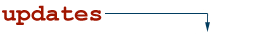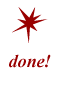# fuzzy logicA type of logic that recognizes more than simple true and false values. With fuzzy logic, propositions can be represented with degrees of truthfulness and falsehood. For example, the statement, "Today is sunny," might be 100 percent true if there are no clouds, 80 percent true if there are a few clouds, 50 percent true if it's hazy, and 0 percent true if it rains all day. Fuzzy logic has proved to be particularly useful in expert systems and other artificial intelligence (AI) applications and is usually used as the underlying logic system for fuzzy expert systems. It is also used in some spell checkers to suggest a list of probable words to replace a misspelled one. Fuzzy logic is a superset of conventional logic (also known as "Boolean logic") that has been extended to handle the concept of partial truth-truth values between "completely true" and "completely false."

Dr. Lotfi Zadeh of UC Berkeley introduced fuzzy logic in the 1960's as a means to model the uncertainty of natural language. Rather than regard fuzzy theory as a single theory, we should regard the process of "fuzzification" as a methodology to generalize any specific theory from a crisp (discrete) form to a continuous (fuzzy) form. Researchers have also introduced fuzzy calculus, fuzzy differential equations, and so on.

NetLingo Classification: Net Technology